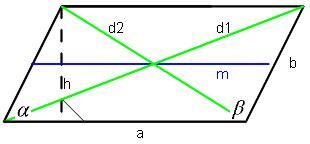Parallelogram

2 opposite sides are parallel and have the same length.
 Side length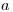= Side length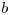= Height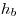= Side length= Side length= Height= Circumfirence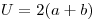= Area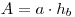= Diagonal 1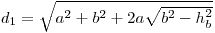= Diagonal 2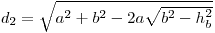= Angle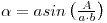= Angle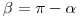=